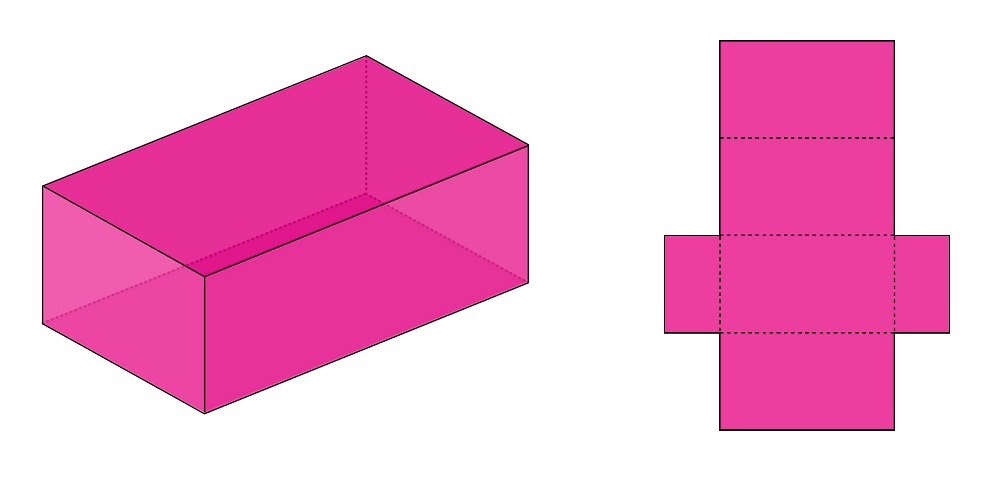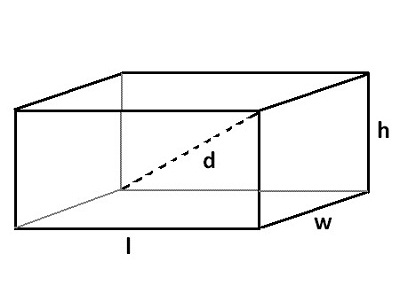# Area of Cuboid Calculator

Cuboid calculator is an online tool to calculate area of cuboid, volume, perimeter and rectangular length of the diagonal. Enter the length, width and height to calculate surface area.

## What is Cuboid ?

A rectangular solid bounded by six rectangular plane faces is called a cuboid. Cuboid – 6 flat faces of which 4 are long (rectangular) and 2 are smaller (square), 12 straight edges of which 4 are longer and 8 are shorter, 8 corners (vertices).### Examples of Cuboid

A match box, a brick, a book, etc., are all examples of a cuboid.

The following are some definitions of terms related to a cuboid:

(i) The space enclosed by a cuboid is called its volume.

(ii) The line joining opposite corners of a cuboid is called its diagonal.
A cuboid has four body diagonals.
A diagonal of a cuboid is the length of the longest rod that can be placed in the cuboid.

(iii) The sum of areas of all the six faces of a cuboid is known as its total surface area.

(iv) The four faces which meet the base of a cuboid are called the lateral faces of the cuboid.

(v) The sum of areas of the four walls of a cuboid is called its lateral surface area.

### Cuboid Formulae

For a cuboid of length = l units, breadth = b units and height = h units, we have:

Sum of lengths of all edge = 4 (l + b + h) units.

Diagonal of cuboid = Sqrt(l2 + b2 + h2)

Total Surface Area of cuboid = 2(lb + bh + lh) sq. units

Lateral Surface Area of a cuboid = Area of four walls of a room = [2h(l + b)]] sq. units

Volume of cuboid = (l × b × h) cubic units

## Latest Blog topics### Spreading Knowledge Across the World

USA - United States of America  Canada  United Kingdom  Australia  New Zealand  South America  Brazil  Portugal  Netherland  South Africa  Ethiopia  Zambia  Singapore  Malaysia  India  China  UAE - Saudi Arabia  Qatar  Oman  Kuwait  Bahrain  Dubai  Israil  England  Scotland  Norway  Ireland  Denmark  France  Spain  Poland  and  many more....